• matlabplot函数 坐标轴标注

千次阅读 2020-02-17 19:45:16
坐标轴标注 https://jingyan.baidu.com/article/da1091fb1770a1027849d692.html
展开全文matlab plot
• 本博文源于matlab基础，用例子引入，大家只需要照猫画虎进行套用即可。 例子：绘制两条曲线y=sin(x+3),y=e^(sin(x))的图形,范围[-2*pi,2*pi] 例子：画出三个函数函数y=2*x,y=cos(x),y=sin(x)，范围[-3,3]并作相应的...

本博文源于matlab基础，用例子引入，大家只需要照猫画虎进行套用即可。

绘制两条曲线y=sin(x+3),y=e^(sin(x))的图形,范围[-2pi,2pi]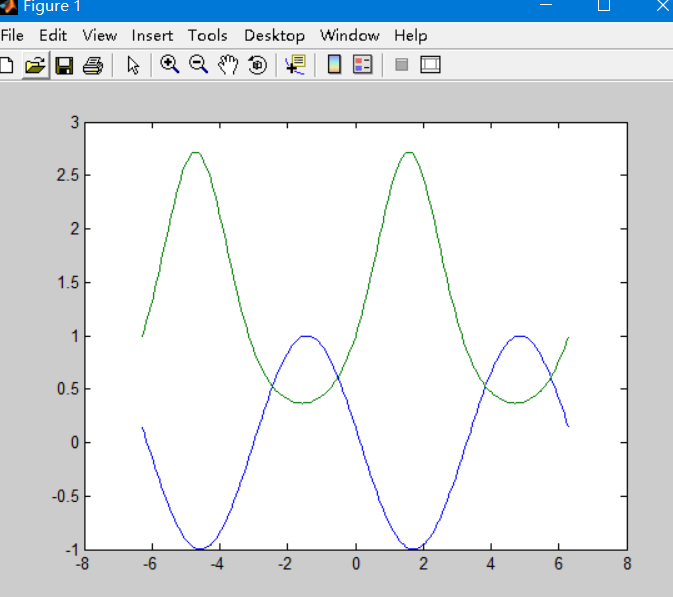这里千万不要直接plot(y)，这样两者作为混合图形，效果既错误又丑陋！

>> x=-2*pi:pi/50:2*pi;
>> y=[sin(x+3);exp(sin(x))];
>> plot(x,y)

画出三个函数函数y=2*x,y=cos(x),y=sin(x)，范围[-3,3]并作相应的图形标注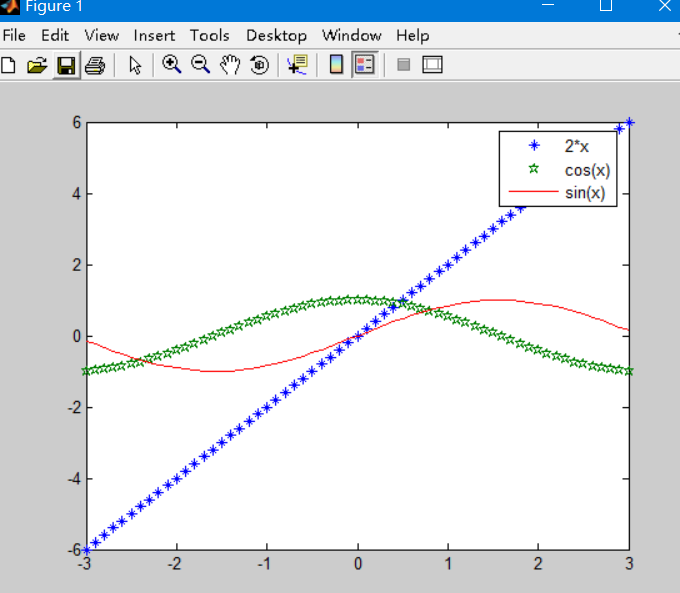>> x=-3:0.1:3;
>> y1=2*x;
>> y2=cos(x);
>> y3=sin(x);
>> plot(x,y1,'*',x,y2,'P',x,y3)
>> legend('2*x','cos(x)','sin(x)')
>>

展开全文matlab 数据可视化
• matlabplot画图

2021-04-24 16:23:34
使用matlab 2014b画图过程中的一些问题记录(是的2020年快2021年了我还在用2014版)1. 线形、标记符和颜色代码 (上图源于网络 https://www.cnblogs.com/sggggr/p/12560428.html 侵删)1.1 举个栗子：figure()x = ...

使用matlab 2014b画图过程中的一些问题记录(是的2020年快2021年了我还在用2014版)

1. 线形、标记符和颜色代码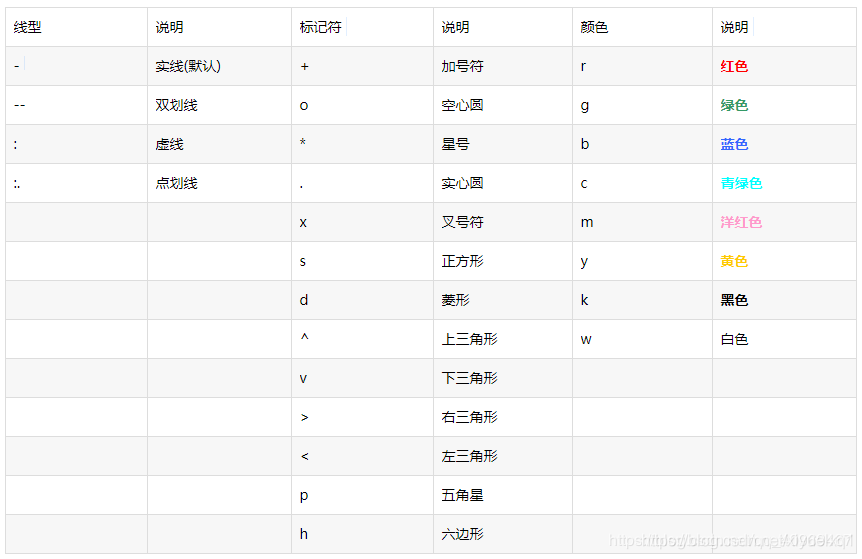(上图源于网络 https://www.cnblogs.com/sggggr/p/12560428.html 侵删)

1.1 举个栗子：

figure()

x = linspace(-2*pi,2*pi);

y = sin(x);

plot(x,y,'--g'); %线型和颜色直接放一起

画图结果：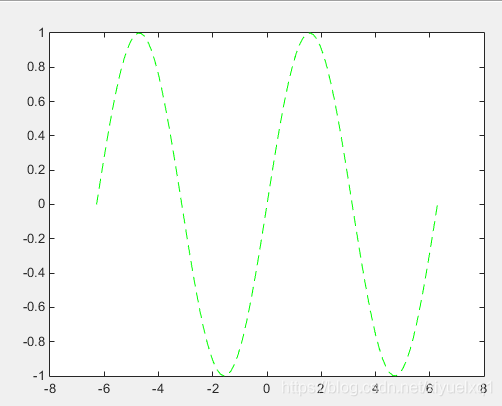1.2 加粗线形——设置LineWidth线宽的取值

plot(x,y,'--g','LineWidth',2);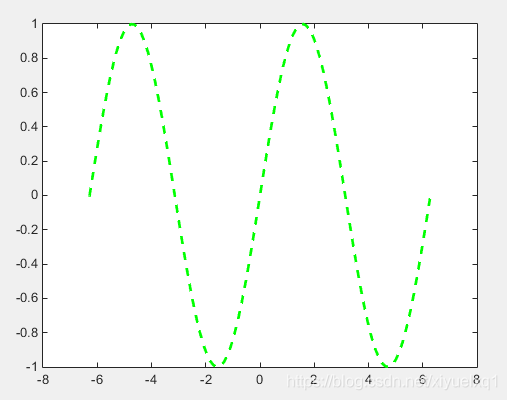1.3 标记x每一点幅值

plot(x,y,'--g','Marker','*'); % Marker不能去掉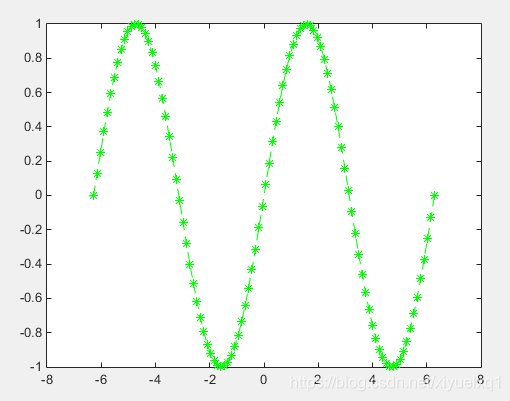2. 坐标轴、图名和网格线

xlabel(); %横坐标

ylabel();% 纵坐标

title(); % 图标题

grid on; % 显示网格线

上面这些都挺简单的，在括号内加要设置的文字即可，但是！

2.1 如需加粗字体

title('\bf{aaaa}'); % \bf对aaaa进行加粗，有没有{}都可以

2.2 如需要换行：

title({'aaaaa';'dddd'}); % 大括号里面需要换行的字段用分号隔开

2.3 如设置字号：

title({'aaaaa';'dddd'},'Fontsize',14);% Fontsize设置字号大小

注：在一次编写标题时，标题字符含有下划线，然后下划线后面的1个字符就变成了下标！如果需要多个字符下标，就在每个字符前面都打上1个下划线。

title('正弦_函_数图像');

效果如下：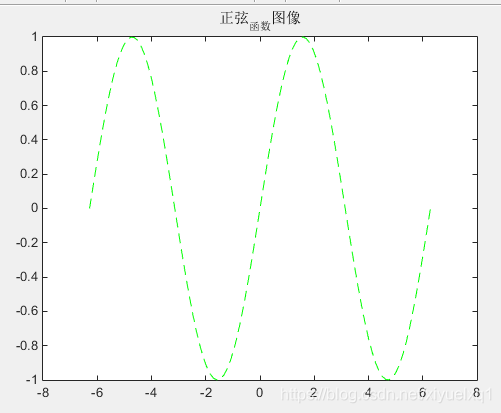如果想打成上标，就把下划线改成^ 就可以；如果并不想文字上下标只是想单纯打出_ 或^ ，暂时还不知道……

3. 一图多线、标注

在同一横坐标画出不同的函数曲线，并对曲线进行标识。

3.1 hold on和 legend 语句

就plot完第一个曲线，后面只加一句 hold on，之后的曲线都可以留在同一个图上，画新的图就重写一个figure()语句！

hold on; % 使得plot多个函数在一个坐标轴内

legend('a','b','c','d'); % 对图中的线在左上角进行标注

figure() ; % 建立新的图，括号里可写正整数表示图的序号

figure()

x = linspace(-2*pi,2*pi);

y1 = sin(x);

y2 = cos(x);

plot(x,y1,'--g','LineWidth',2); %线性和颜色直接放一起

hold on;

plot(x,y2,'r','LineWidth',2);

legend('y1','y2');

或者直接一个plot函数画多条线(效果一样)：

figure()

x = linspace(-2*pi,2*pi);

y1 = sin(x);

y2 = cos(x);

plot(x,y1,'--g',x,y2,'r','LineWidth',2);

legend('y1','y2');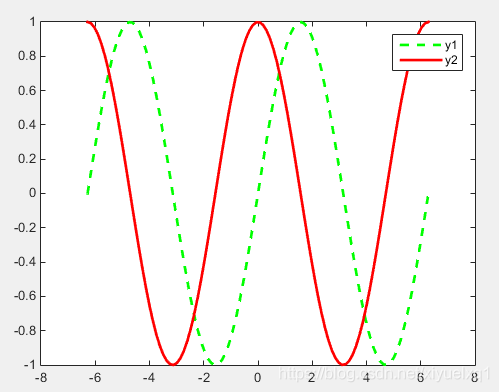3.2 text() 和 gtext() 语句

有时候如果图片需要黑白打印，颜色不方便进行分辨，想要更直观的知道哪条曲线是什么而不使用图注去一一对应，可以直接在图中指定坐标处对曲线进行标识。

3.2.1 text() 直接在坐标点处加文字

text(pi+0.3,0,'\bfy1'); % 在坐标(pi+0.3,0)处加上标识文本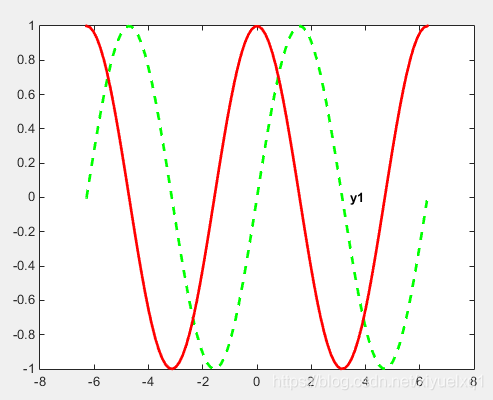3.2.2 gtext()在运行后在鼠标选定位置添加文字

gtext('\bfy2'); % 运行后鼠标点击就直接把文本放在那里了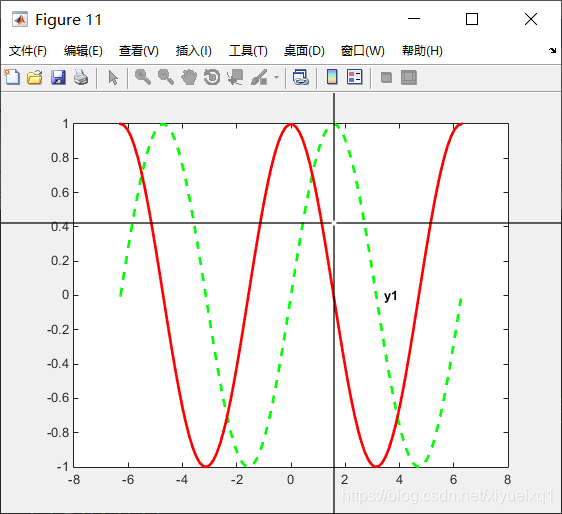完美标记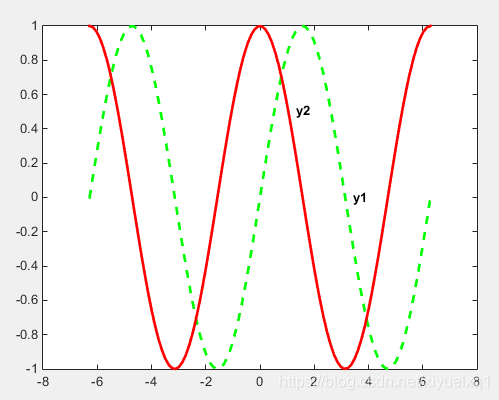4. 更改坐标图外围颜色

set(gcf, 'Color', [0.5,0.5,0]); % []内是r,g,b数值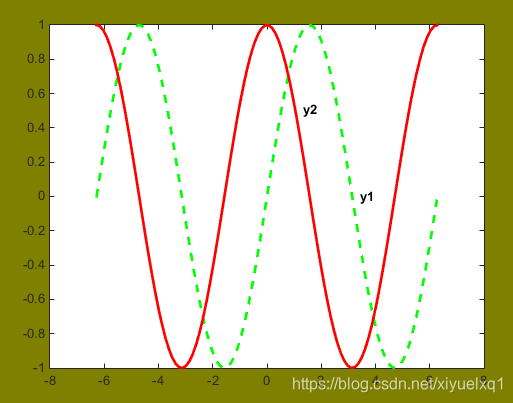后面持续更新……

原文链接:https://blog.csdn.net/xiyuelxq1/article/details/110567043

展开全文• y=x.*exp(-x)plot(x,y),xlabel('x'),ylabel('y'),title('y=x*exp(-x)')然后我在command窗口中依次输入：>> x=0:0.1:1x =0 0.1000 0.2000 0.3000 0.4000 0.5000 0.6000 0.7000 0.8000 0.9000...

y=x.*exp(-x)

plot(x,y),xlabel('x'),ylabel('y'),title('y=x*exp(-x)')

然后我在command窗口中依次输入：

>> x=0:0.1:1

x =

0    0.1000    0.2000    0.3000    0.4000    0.5000    0.6000    0.7000    0.8000    0.9000    1.0000

>> y=x.*exp(-x)

y =

0    0.0905    0.1637    0.2222    0.2681    0.3033    0.3293    0.3476    0.3595    0.3659    0.3679

>> plot(x,y),xlable('x'),ylable('y'),title('y=x*exp(-x)')

??? Undefined function or method 'xlable' for input arguments of type 'char'.

此时已经弹出结果所对应的图了，但是为什么报错了呢？

这不图都出来了，丫的matlab咱还嚷嚷呢？！

还好，这次没放过，觉得还是哪里不对，赶紧在命令行键入如下指令：

>> help xlable

是不matlab出问题了，然后直接打开GUI上的help窗口，输入xlable，居然“No Matches Found”！？越来越不对劲儿了，baidu了一下(不是打广告滴)，网上果然还有一样错误的(http://zhidao.baidu.com/question/75740085.html)，^_^

别人的错误如下：

请问我的matlab为什么用不了xlable函数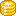悬赏分：10 - 解决时间：2008-11-16 12:56

以下为运行的程序：x=0:0.1:2*pi;

>> plot(x,sin(x))

>> hold on

>> plot(x,cos(x),'ro')

>> title('y1=sin(x),y2=cos(x)')

>> xlable('x')

??? Undefined function or method 'xlable' for input arguments of type 'char'.

跪求好心人指点，在哪里下xlable函数？是哪个工具箱的啊？”

回答是：

"xlabel('x')

你的最后两个字母倒了。

"

看了半天，什么最后两个字母颠倒了啊？？！！然后仔细对比xlabel('x')所有的字母，终于发现原来xlable应该是xlabel！不是吧，我e文好像没这烂呢！赶紧打开金山词霸，输入lable，太让我失望了：

“lable

抱歉！本地没有该词条解释，您可以选择以下方式：

请到网络查询

给出拼写近似的单词

加入用户词典”

再输入label，“label

[5leibl]

n.

标签, 签条, 商标, 标志

vt.

贴标签于, 指...为, 分类, 标注

[计] 加上或修改磁盘的标签”

这个结果也太让人颠覆了，原来这么多年，一直活在label的世界里！！！

还好还好！大小考试好像都没考过这个词的写法，o(∩_∩)o...哈哈

小小xlabel也给我上了深刻的一课！

此label非彼lable也！

label label label...

展开全文• Matlab绘图------图形标注

万次阅读 2019-07-14 10:14:18
在图形中进行详细的标注时，需要用到两个函数，一个是text，一个是gtext,它们均可以在图形中具体位置进行标注， text函数可以在图形中的指定位置进行标注，以此用来显示图形的某一些特殊位置的变化情况； ...Matlab图形标注
• matlabplot基本用法

千次阅读 2021-04-18 08:21:53
plot(y)生成的图形是以序号为横坐标、数组y的数值为纵坐标画出的折线。//////////////////////////////////////////////////////////////////////////////////////////////////>> x=linsp...
• x=1:1:150; y = 1:1:150; maker_idx = 1:5:150; plot(x,y,'-^','MarkerIndices',maker_idx)matlab
• 您可以使用viscircles(这需要Image Processing Toolbox)轻松完成此操作,但我不认为输出...dots = plot(timeline, Origdata, '.');hold onfor k = 1:numel(dots)plotdata = get(dots(k));centers = [plotdata.XData(...
• 数据点画圈： 实线： plot(time1,'o-'); 结果： 虚线： plot(time1,'--o'); 结果： 画线换颜色 plot(time1,'o--r'); 结果：matlab
• MATLAB中标注图例

千次阅读 2021-04-18 15:03:02
当在一幅图中出现多种图形中，用户可以根据自己的需要，... >> plot(x, y1, '-r', x, y2, '+b', x, y3, '*g') >> xlabel('xValue'), ylabel('yValue') >> axis([0, 7, -2 ,3]) >> legend('sin(x)', 'x/2', 'cos(x)') >>
• 一、plot 函数绘制多个图形、 二、legend 函数标注图形、 三、图形修饰、matlab plot legend
• Matlab绘图坐标轴的设置教程

千次阅读 2021-04-18 09:44:48
编程，绘图，设置坐标轴，做出自己的坐标轴1. axis([xmin xmax ymin ymax])设置当前图形的坐标范围，分别为x轴的最小、最大值，y轴的最小最大值2. V=axis返回包含当前坐标范围的一个行向量3. axis auto将坐标轴刻度...
• 这个需求看起来很拗口，不过也是...另外关于颜色设置，由于目前matlab可以自己选择一些人类看起来舒服、区分度较高的颜色，我已经很久不自己指定曲线颜色了。搜了一下，发现并不是我一个人有标题中的需求，例如这里： ...
• plot(y) 生成的图形是以序号为横坐标、数组y的数值为纵坐标画出的折线。>> x=linspace(0,2*pi,30); % 生成一组线性等距的数值>> y=sin(x);>> plot(x,y) 生成的图形是上30个点连成的光滑的正弦...
• 本文主要介绍了Matlabplot基本用法的具体使用，分享给大家，具体如下：>> y=[0 0.58 0.70 0.95 0.83 0.25];>> plot(y)生成的图形是以序号为横坐标、数组y的数值为纵坐标画出的线>> x=linspace(0,2*pi,30); % 生成...
• plot(y)生成的图形是以序号为横坐标、数组y的数值为纵坐标画出的折线。//////////////////////////////////////////////////////////////////////////////////////////////////>>x=linsp...
• MATLAB图形标注

千次阅读 2019-01-18 19:19:48
如果在我们创建了句柄，那么我们在MATLAB主界面的右侧，双击句柄，可以打开句柄属性，在里面也可以查看和修改属性。 下面介绍一些常用的代码修改方式，优点是比较快捷。 线条属性 属性的设置基本有三种方法，...MATLAB 注释
• plot(x,sin(x),'-k', 'LineWidth',4); set(gca,'linewidth',1,'fontsize',20,'fontname','Times'); %依次设置坐标轴的属性分别为：坐标轴的线宽（2），坐标轴的刻度字号大小（20），坐标轴的刻度字体（罗马体）。 ...
• 得出图形如图： 想要得到的图像如图： 即在legend标注栏里只要标注曲线，而不把点的标注标出来。 QQ截图未命名.jpg (43.84 KB, 下载次数: 10) 2008-5-27 07:07 上传 QQ截图未命名2.jpg (56.1 KB, 下载次数: 10) ...
• matlab中二维线画图函数 1. plot(x,y); %x y 为相应点集 2.plot(x,y1,x,y2); % 在一个窗口下绘制多条曲线之方法一 3.hold on %在一个窗口下绘制多条曲线之方法二 plot(x,y1); plot(x,y2); hold off 4.plot...
• 也就是说，使用plot 函数之前，必须首先定义好曲线上每一点的x 及y 坐标，常用格式为：1、二维数据曲线图指数函数和余弦函数之间用点乘运算.*plot函数，plot(x,y)具有两个纵坐标标度的图像，可以使用plotyy(x1,y1,x2...
• Matlab plotplot绘制图形时添加图形标记的标记符如下： 标记符 点形 + 加号 o 圆圈 * 星号 . 实心点 x 叉号 s 正方形 d 钻石形 ^ 上三角形 v 下...
• 添加中文字体到MATLAB的文件夹：D:\Program Files\MATLAB\R2011a\sys\java\jre\win32\jre\lib\fonts\ 2. 然后在画图的时候需要添加text的位置直接指定你添加的中文字体title(‘Test’,’FontName’,’STFangSong...matlab plot
• 在MATLAB中的图例标注及实例说明

万次阅读 多人点赞 2017-10-19 21:33:40
plot(Y)：其中输入参数Y就是Y轴的数据，一般习惯性输入向量 plot(X1,Y1,LineSpec,...,Xn,Yn,LineSpec):LineSpec为选项（开关量）字符串，用于设置曲线颜色、线型、数据点等；LineSpec的标准设定值见表1，前7种颜色...MATLAB 信道容量 图例标注 实例
• x = -pi:pi/10:pi; y = tan(sin(x)) - sin(tan(x));...plot(x,y,'--gs',... 'MarkerIndices',[find(y<-2),find(y>2)],... 'LineWidth',2,... 'MarkerSize',10,... 'MarkerEdgeColor','r',... ...
• Matlab中，除了plot绘制曲线的一般属性外，还有曲线线宽、标记点大小、标记点边框颜色和填充颜色等属性。这些需要通过plot(...，'PropertyName',ProperValue,...)这样的语法格式来实现。​其中可供选择的ProperName...
• figure(2) plot(h2,kr,'LineWidth',2) hold on plot(thickness,kr1,'r*') ...text(1.65,1.1,' \leftarrow skin depth') %文字标注 xlabel('thickness(mm)') ylabel('ratio AC to DC ') legend('Analyze','FEM') % titl.matlab
• 下面的代码是一个简单的批量标注legend的例子，R,G,B三行是用来控制颜色的，随便弄的，颜色的话要好看还得再调整clear all;clc;baseString = 'R';r = 1:10;legendString = cell(1,length(r));for i = 1:length(r)tmp......

matlabplot标注matlab 订阅